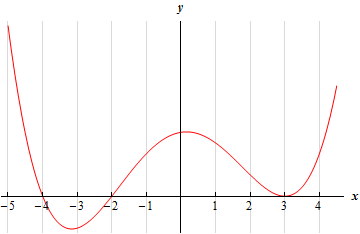Paul's Online Notes
Home / Calculus I / Applications of Derivatives / The Shape of a Graph, Part II
Show Mobile Notice Show All Notes Hide All Notes
Mobile Notice
You appear to be on a device with a "narrow" screen width (i.e. you are probably on a mobile phone). Due to the nature of the mathematics on this site it is best views in landscape mode. If your device is not in landscape mode many of the equations will run off the side of your device (should be able to scroll to see them) and some of the menu items will be cut off due to the narrow screen width.

### Section 4.6 : The Shape of a Graph, Part II

2. Below is the graph the 2nd derivative of a function. From this graph determine the intervals in which the function is concave up and concave down.Hint : Be careful with this problem. The graph is of the 2nd derivative of the function and so we don’t just write down intervals where the graph is concave up and concave down. Recall how the 2nd derivative tells us where the function is concave up and concave down and this problem is not too bad.
Show Solution

We need to be careful and not do this problem as we did the first practice problem. The graph given is the graph of the 2nd derivative and not the graph of the function. Therefore, the answer is not just where the graph is concave up or concave down.

What we need to do here is to recall that if the 2nd derivative is positive (i.e. the graph is above the $$x$$-axis) then the function in concave up and if the 2nd derivative is negative (i.e. the graph is below the $$x$$-axis) then the function is concave down.

So, it is fairly clear where the graph is above/below the $$x$$-axis and so we have the following intervals of concave up/concave down.

$\require{bbox} \bbox[2pt,border:1px solid black]{{{\mbox{Concave Up : }}\left( { - \infty , - 4} \right),\left( { - 2,3} \right)\,\,\,\& \,\,\,\left( {3,\infty } \right)\hspace{0.25in}\,\,\,\,\,\,\,\,\,\,\,\,\,\,\,{\mbox{Concave Down : }}\,\,\left( { - 4, - 2} \right)}}$

Even though the problem didn’t ask for it we can also identify that $$x = - 4$$ and $$x = - 2$$ are inflection points because at these points the concavity changes. Note that $$x = 3$$ is not an inflection point. Clearly the 2nd derivative is zero here, but the concavity doesn’t change at this point and so it is not an inflection point. Keep in mind inflection points are only where the concavity changes and not simply where the 2nd derivative is zero.